# R S Aggarwal Solutions for Class 11 Maths Chapter 28 Differentiation Exercise 28D

Exercise 28D of Chapter 28 is about derivative of the quotient of two functions, which states that, if u and v are two differentiable functions and v not equal to zero, then u/v is also differentiable, and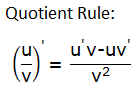Class 11 Maths Chapter 28 Differentiation Exercise 28D is based on the topic: Derivative of the quotient of two functions. Learn how to find the derivative of functions in the form f(x)/g(x), through R S Aggarwal Solutions that are provided in this section.

## Download PDF of R S Aggarwal Solutions for Class 11 Maths Chapter 28 Differentiation Exercise 28D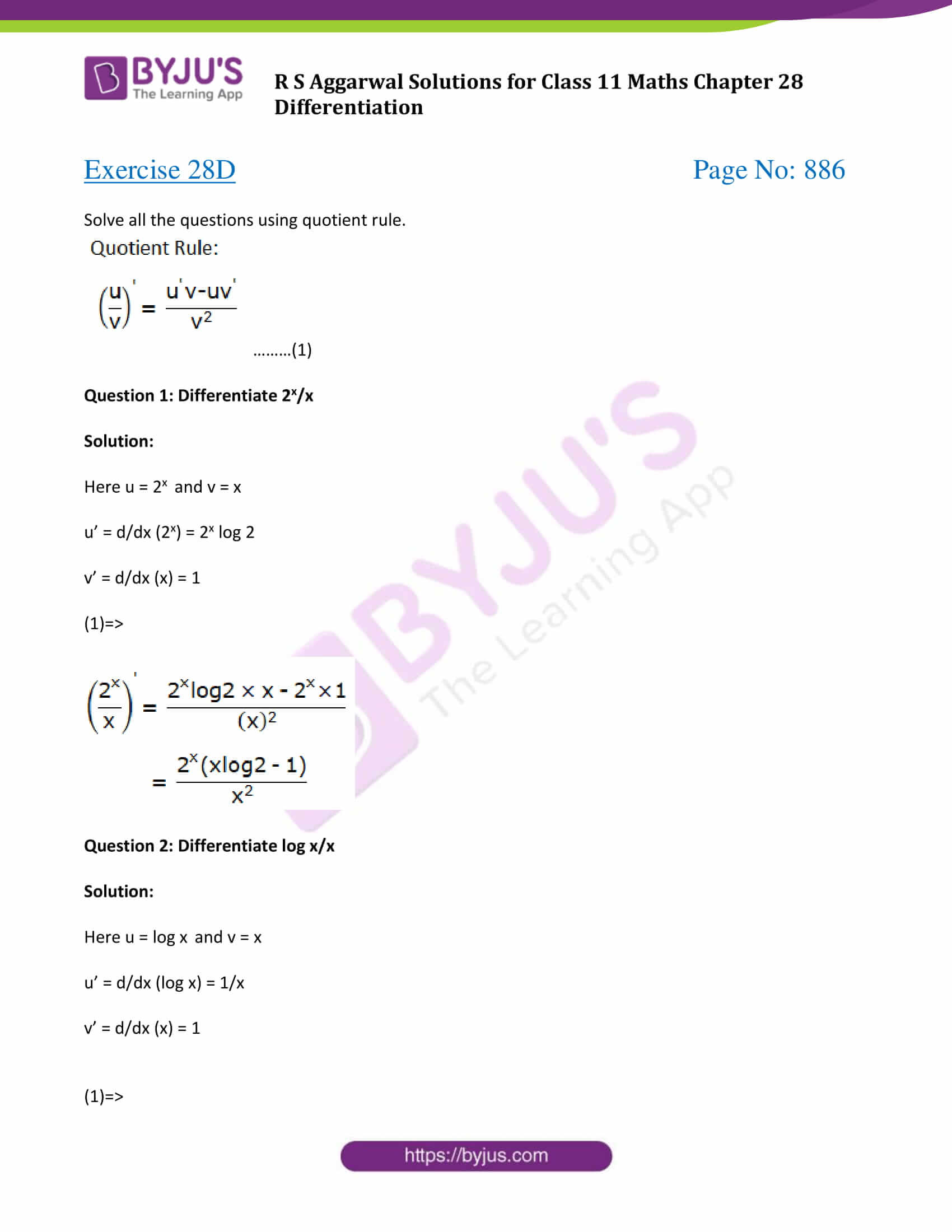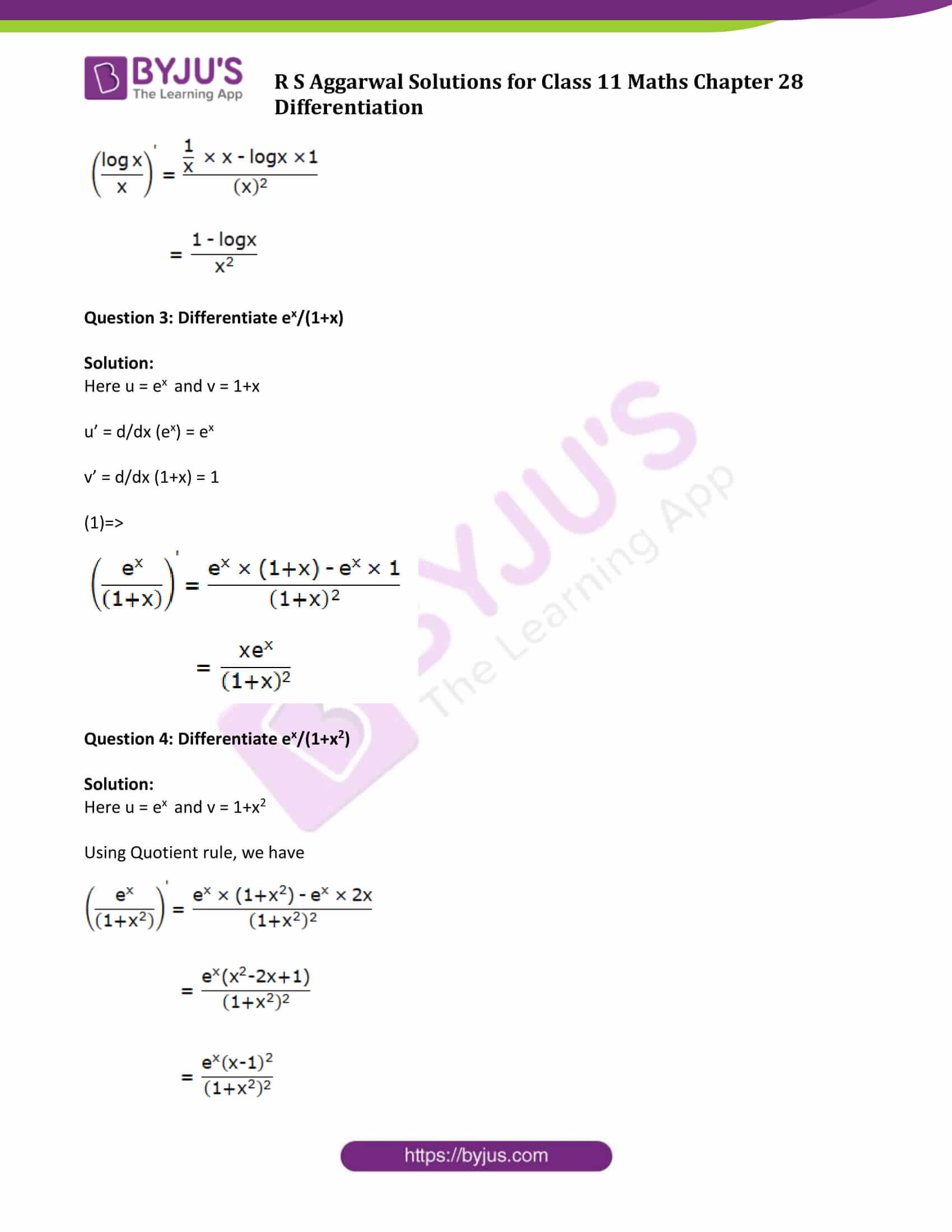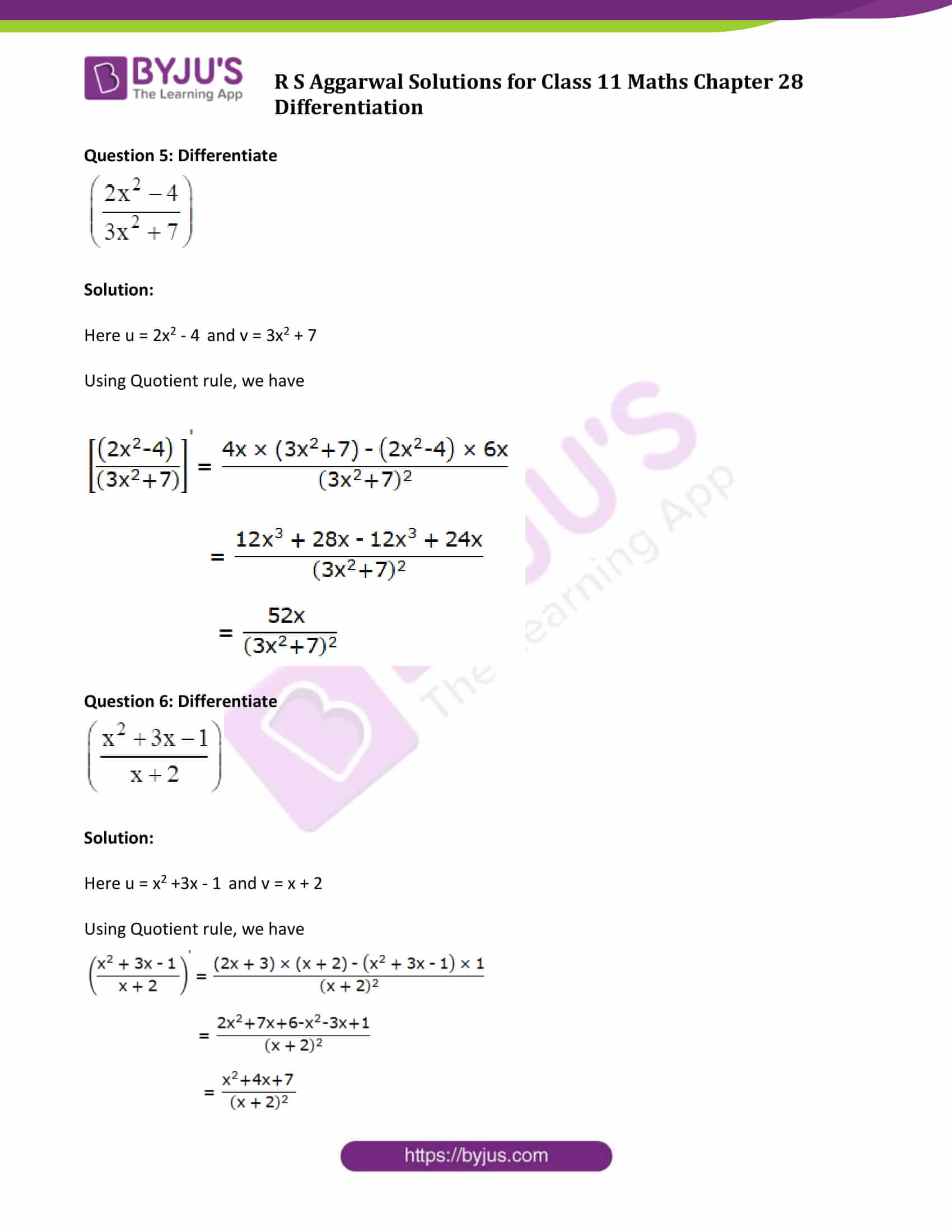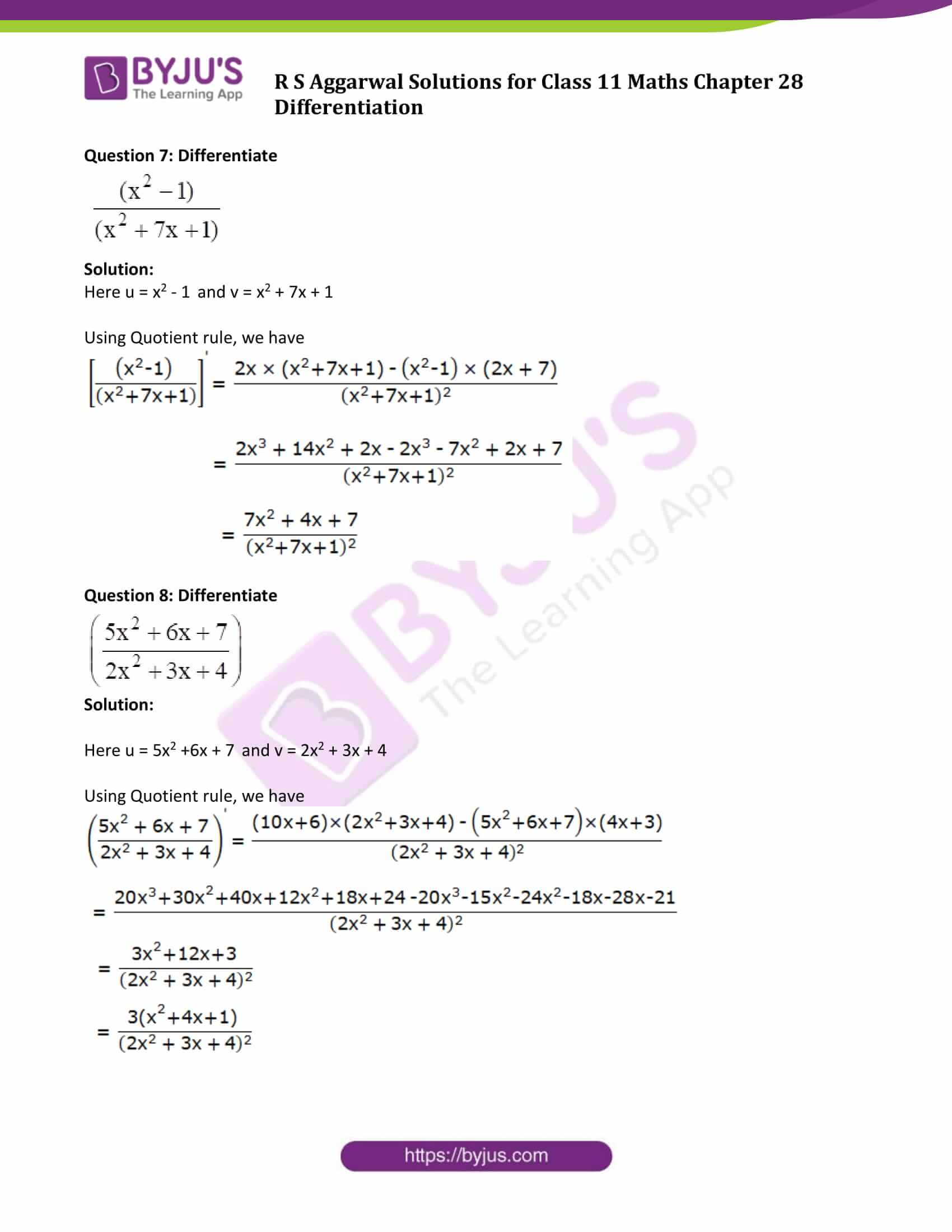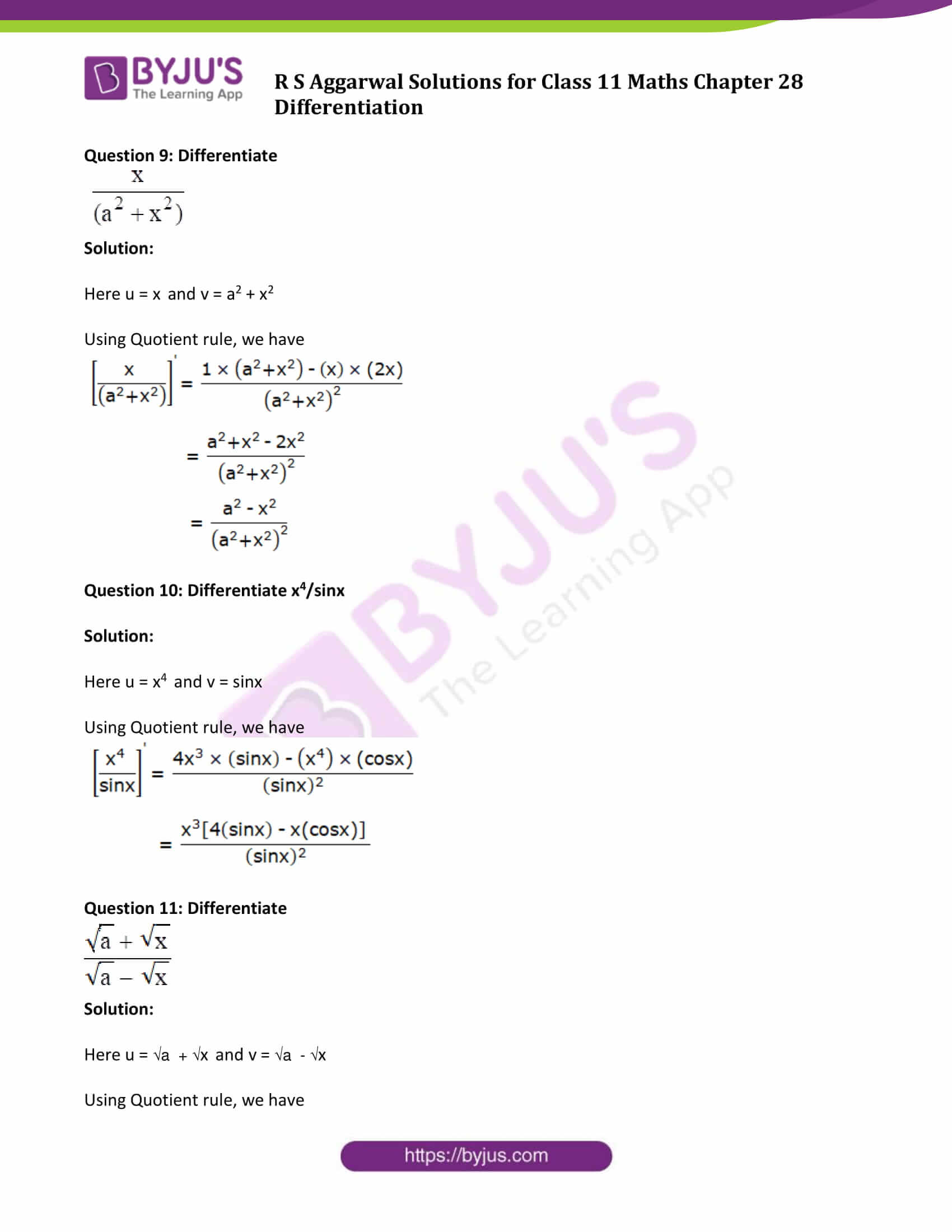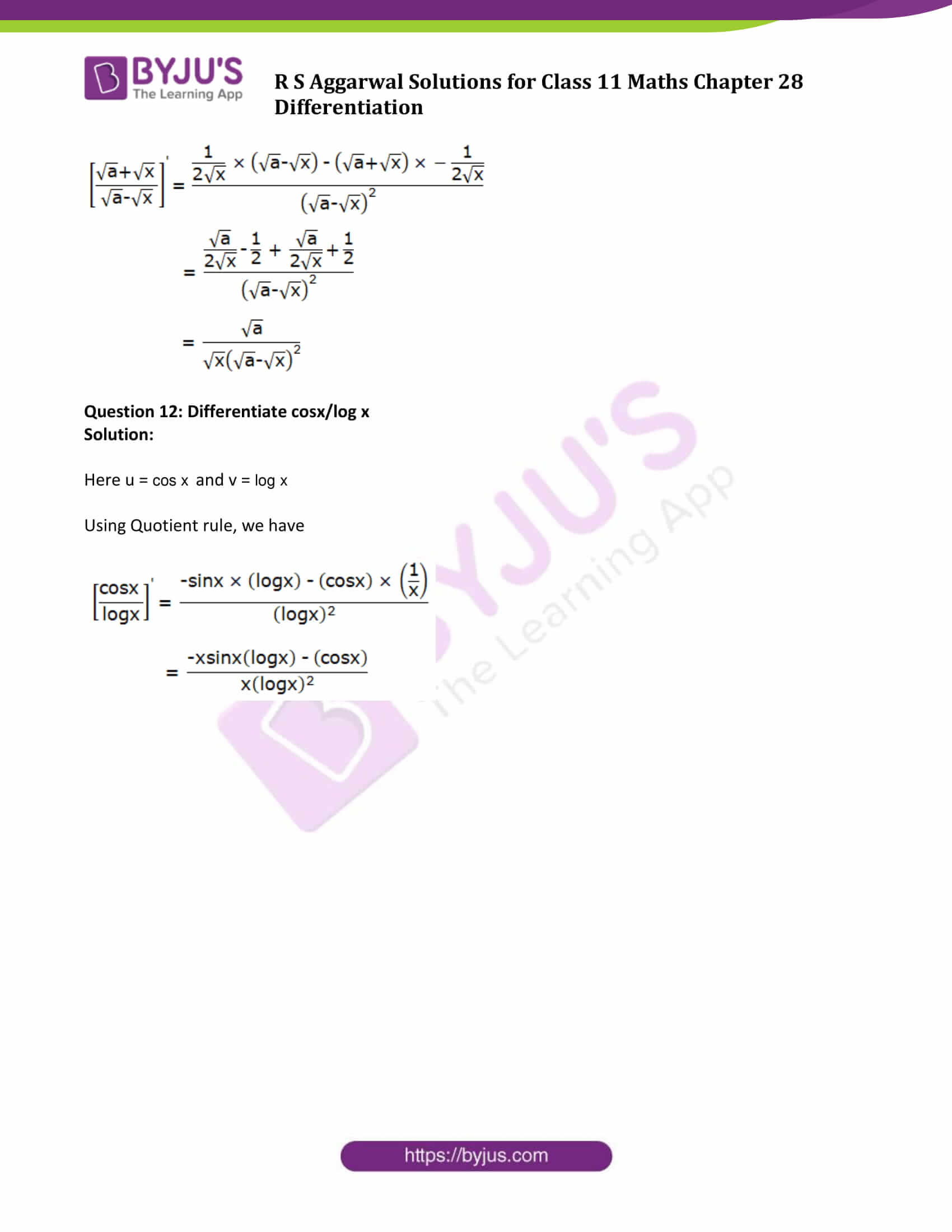### Access Answers to Maths R S Aggarwal Class 11 Chapter 28 Differentiation Exercise 28D Page number 886

Solve all the questions using quotient rule.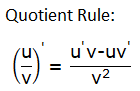………(1)

Question 1: Differentiate 2x/x

Solution:

Here u = 2x and v = x

u’ = d/dx (2x) = 2x log 2

v’ = d/dx (x) = 1

(1)=>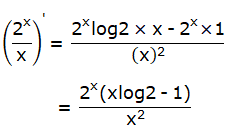Question 2: Differentiate log x/x

Solution:

Here u = log x and v = x

u’ = d/dx (log x) = 1/x

v’ = d/dx (x) = 1

(1)=>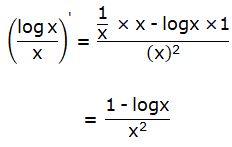Question 3: Differentiate ex/(1+x)

Solution:

Here u = ex and v = 1+x

u’ = d/dx (ex) = ex

v’ = d/dx (1+x) = 1

(1)=>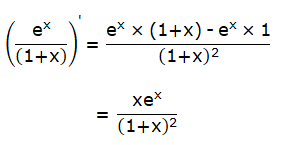Question 4: Differentiate ex/(1+x2)

Solution:

Here u = ex and v = 1+x2

Using Quotient rule, we have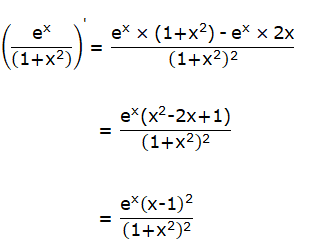Question 5: Differentiate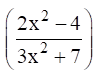Solution:

Here u = 2x2 – 4 and v = 3x2 + 7

Using Quotient rule, we have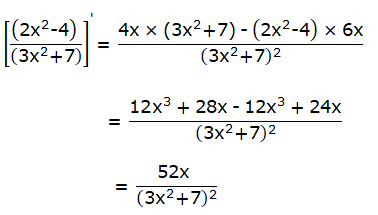Question 6: Differentiate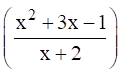Solution:

Here u = x2 +3x – 1 and v = x + 2

Using Quotient rule, we have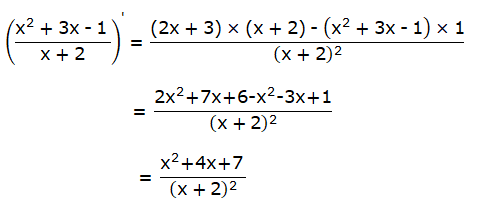Question 7: Differentiate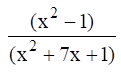Solution:

Here u = x2 – 1 and v = x2 + 7x + 1

Using Quotient rule, we have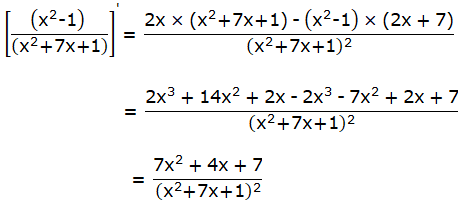Question 8: Differentiate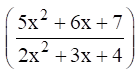Solution:

Here u = 5x2 +6x + 7 and v = 2x2 + 3x + 4

Using Quotient rule, we haveQuestion 9: Differentiate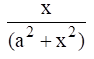Solution:

Here u = x and v = a2 + x2

Using Quotient rule, we have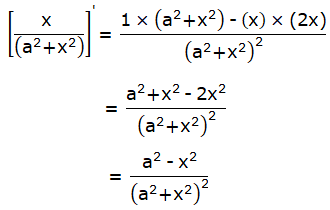Question 10: Differentiate x4/sinx

Solution:

Here u = x4 and v = sinx

Using Quotient rule, we have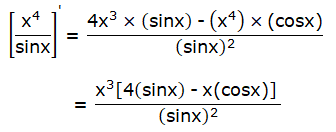Question 11: Differentiate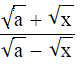Solution:

Here u = √a + √x and v = √a – √x

Using Quotient rule, we have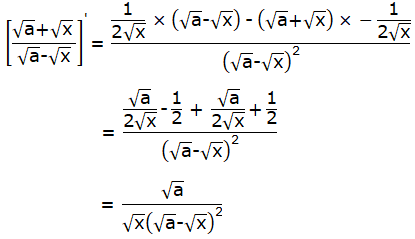Question 12: Differentiate cosx/log x

Solution:

Here u = cos x and v = log x

Using Quotient rule, we have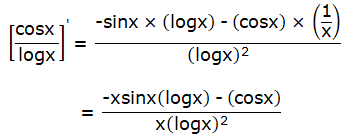## Access other exercise solutions of Class 11 Maths Chapter 28 Differentiation

Exercise 28A Solutions

Exercise 28B Solutions

Exercise 28C Solutions

Exercise 28E Solutions